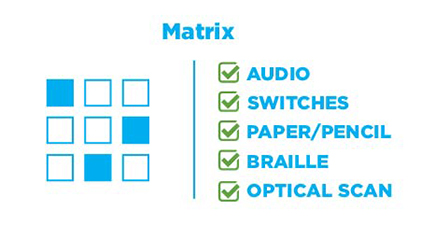# Matrix Labeling

Matrix labeling items contain a grid in which elements are in rows, and the labels are column headers. Depending on the item, labels may be used more than once. Users select a label for each element by filling in answer bubbles at the intersections of rows and columns.1. Select the x value that makes each equation true.
2.  x = -3 x = -2 x = -4 x = -1 x2 – x – 6 = 03x2 – 12x – 15 = 06x2 – 6x – 72 = 06x2 + 18x – 24 = 0